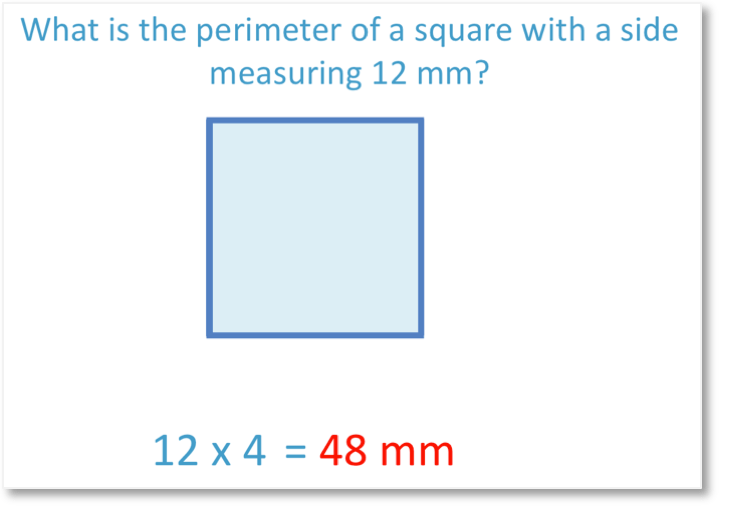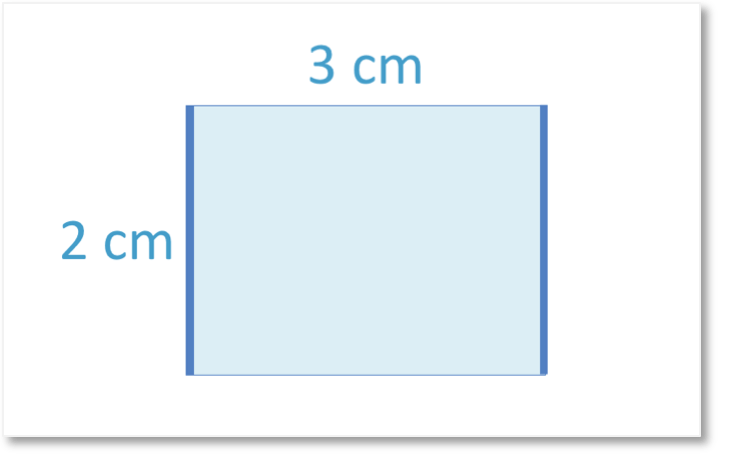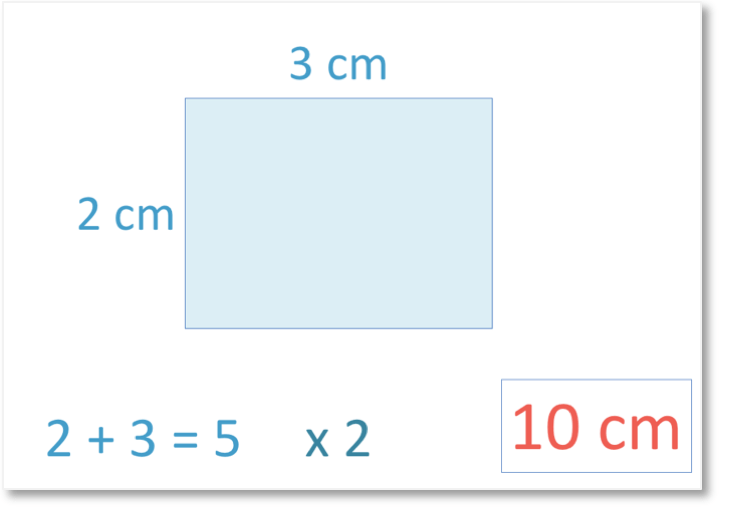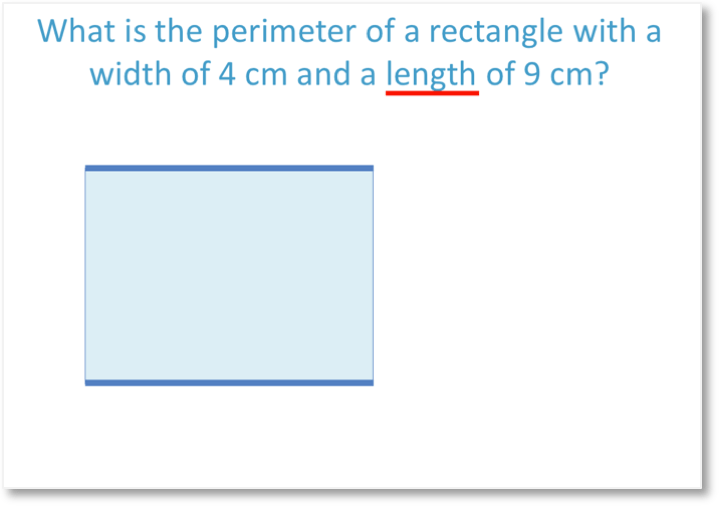# How to Find the Perimeter of Rectangles and Squares

How to Find the Perimeter of Rectangles and Squares• The perimeter is the total distance around the outside of a shape.
• To find the perimeter of a shape, we add the sides up.
• The opposite sides of a rectangle have the same length.
• Adding all four sides we have 2 + 3 + 2 + 3 = 10 cm.
• The perimeter of the rectangle is 10 cm.
• We can also find the perimeter of the rectangle by adding the two adjacent sides and then doubling the answer.
• 2 + 3 = 5cm.
• Doubling this gives us a perimeter of 10 cm.
• The total distance around the outside of the rectangle is 10 cm.

Add all four sides of the rectangle to find its perimeter.• The perimeter is the total distance around the outside of the square.
• On a square all four sides are the same length.
• Each side is 12 mm long.
• There are four sides so we have four lots of 12 mm.
• 4 × 12 mm = 48 mm.
• The perimeter of the square is 48 mm.
• To find the perimeter of a square, multiply the length of one side by 4.# The Perimeter of Rectangles and Squares

## How to Find the Perimeter of a Rectangle

To find the perimeter of a rectangle add up the lengths of all four sides. Alternatively, add the length and width of the rectangle together and then double this result.

Perimeter is the total distance around the outside of a shape. It is found by adding up all of the outside lengths of a shape.

The units of perimeter are lengths. Here are some of the most common units that perimeter is measured in.Here is an example of finding the perimeter of a rectangle when only given 2 of its sides. We are told the length and the width of this rectangle.

The length is 3cm and the width is 2 cm.The opposite sides of a rectangle are the same length.

Therefore if the left side of the rectangle is 2 cm, then so is the right side.If the top side of the rectangle is 3 cm, then so is the bottom side.Once the lengths of all of the sides of a shape are labelled, the perimeter can be found by adding these lengths together.2 + 3 + 2 + 3 = 10

Therefore the perimeter of this rectangle is 10 cm. This means that the total distance around the outside of the shape is 10 cm.

There is a quicker method for working out the perimeter of a rectangle when given the length and the width. To find the perimeter of a rectangle, simply add the length and the width together and then multiply this result by 2.

This is because there are two widths, which are marked below.And two lengths, which are marked below.There are two lengths and two widths on a rectangle. We can add the length and width together and then double this result because there are two of them.

Adding the length and the width, we have 2 + 3 = 5 cm.Since there are two lengths and two widths, we double this to get 10 cm.The formula for the perimeter of a rectangle is Perimeter = 2 × (length + width). This can be written as P = 2(l + w). Simply add the length and width togther and then multiply the result by 2.

The formula for the perimeter of a rectangle can alternatively be written as P = 2l + 2w or P = l + l + w + w.

Here is another example of finding the perimeter of a rectangle. We will use the formula to find the perimeter of this rectangle.P = 2(l + w). We add the length and width and then double it.

20 + 15 = 35 mm. We double this to get the perimeter of 70 mm.

The total distance around the outside of this rectangle is 70 mm.

## Perimeter of Rectangles Word Problems

To solve word problems with perimeter, first draw the problem to visualise it better. Then mark the length of all sides before adding them together.

Here is an example of a word problem for the perimeter of a rectangle.We draw a diagram of the situation to help us visualise it and to mark the lengths on. The width is shown below as the two shorter sides.This is the length. The length is always the longest side.Finding the perimeter of the rectangle, we add all four sides together. We have 4 + 4 + 9 + 9.

We can add the two fours to get eight and we can add the two nines to get 18.

8 + 18 = 26 cm and so, the perimeter of the rectangle is 26 cm.Again we can use the formula to calculate the perimeter of the rectangle more quickly.

P = 2(l + w) and so, P = 2(4 + 9).

P = 2 × 13

P = 26 cm

## How to Find the Perimeter of a Square

To find the perimeter of a square, multiply the length of one side by 4.

The formula for the perimeter of a square is P = 4l, where l is the length of one side of the square.

A square is a shape that has all four sides that are the same length.

Here is a word problem for finding the perimeter of a square.To solve the word problem, we first draw the shape and mark any known sides.

We know that the square has a side length of 12 mm.Since each side is the same length, all four sides are 12 mm long.

We have four lots of 12 mm.

The perimeter is 48 mm.Now try our lesson on Perimeter of Irregular Shapes where we learn how to calculate the perimeter of a variety of different shapes.error: Content is protected !!